Practice Tan, Cot, Sec, Csc Graphs Terms of Use    Contact Person: Donna Roberts1.
Determine an equation for this graph: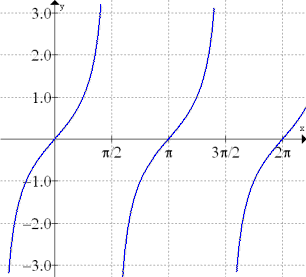Choose:
 y = csc(x) y = sec(x) y = tan(x) y = cot(x)

2.
Determine an equation for this graph: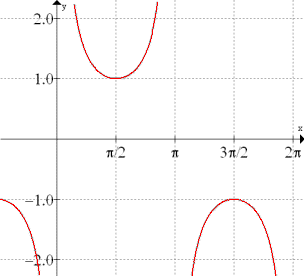Choose:
 y = csc(x) y = sec(x) y = tan(x) y = cot(x)

3.
Determine an equation for this graph: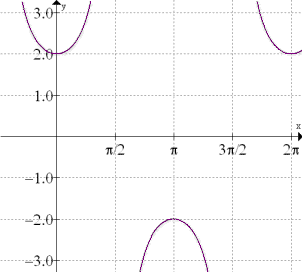Choose:
 y = sec(x) y = 2sec(x) y =2csc(x) y = csc(2x)

4.
Determine an equation for this graph: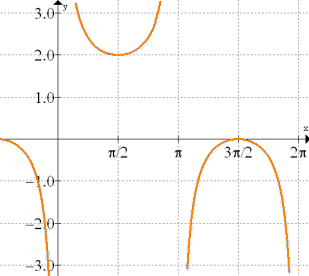Choose:
 y = 2csc(x) y = csc(x) + 1 y = 2csc(x) + 1 y = csc(x) + 2

5.
Determine an equation for this graph: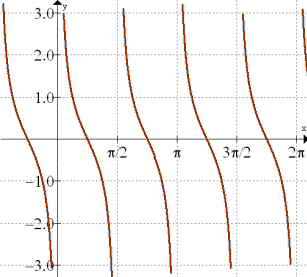Choose:
 y = tan(2x) y = 2tan(x) y = cot(2x) y = 2cot(x)